CipherJan 14, 2017

Part 2: Cube of Saturn and more

Continuation of https://nasanumerology.blogspot.rs/2017/01/saturn-roman-numerals-connection-to.htmlThis is very interesting because 42nd triangular number is 903 - Saturn = 93
"Law of Saturn" = 42 (Reduced), "Freemason" = 42 (R) , "Zionism" = 42 (R)

The cube that Jews wear on their forehead is called 'Tefillin'
"Tefillin" = 2+5+6+9+3+3+9+5 = 42 (Reduced)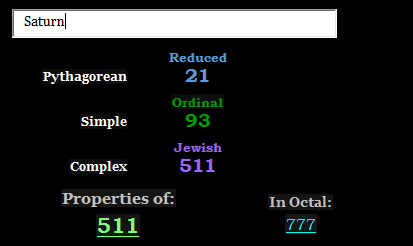God of the Hebrew Bible is called ELohim, he is referred to by many names and all of the names contain EL (Aleph-lamed)
EL was a Canaanite God,  the Canaanites were strongly influenced by Egyptian and Mesopotamian mythology, EL was the supreme God, creator of Heavens and Earth, in the bible he is identified as the God Yahweh3rd on this picture you see - El Shaddai - the part Shaddai is written with 3 letters, Shin-Daleth-Yod = 300+4+10 = 314 (Pi)

Lamed is also the tallest letter of the Hebrew alphabet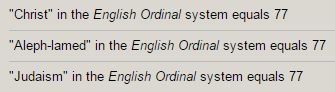Think about how L and J are opposite and think about ELohim sending Jesus to be sacrificed? On unfolded cube?J is curved and facing away from L, it looks like a fish hook and Jesus has a miraculous catch of 'Fish' - 42/33, he is also referred to as a 'Fisher of Men', word "Fisherman" = 93, like Saturn

L is completely straight with a right angle, facing right, word Law starts with L for a good reason, but there are interesting words that start with EL, ELite, ELevation(930 sumerian), ELements(93), ELectricity, interesting that obELisk has L in the middle, L is upright looking like an obelisk

"Obelisk" = 73 (Ordinal) - 21st prime number (Saturn = 21, "Dice" = 21 (Ordinal), "Cube" = 210 (Jewish)

is also upside down - we have 6 numbers on a die, giving us 777 and - 6+1 = 7, 5+2 = 7, 4+3 = 7 - 777
6th triangular number is 21 (Saturn = 21)

7th prime number is 17 (God = 17, EL = 17)
7x17 = 119 (Saturn = 119 F.B. , God Saturn = 119 Ordinal)
7x7 = 49 (227 is 49th prime number)
7x7x7 = 343 (octal of 227) - 227 is closest approximation of Pi with small numbers
"Seven hundred seventy seven" = 314, "Twenty two divided by seven" = 314
Saturn being the God of Time is definitely expected to be in charge of all the cycles (Cycle = 21, Moon = 21 = 7+7+7)

119 and 227 are very connected numbers that come up a lot with Saturn study
119+191+911 = 1221
227+272+722 = 1221
329th prime number is 2207 (329 is 511 in octal system) - Saturn = 511

329+392+239+293+923+932 = 3108 - "Inverse Pi"

"Monument" = 115 (Ordinal) -  Saturn = 511, converting 77 to Octal numbering system you find 115, if you convert the reflection you get 777

Aleph-lamed would have Hebrew Gematria of 31 - which is interesting because of a lot of 13/31's connected to Saturn
31st triangular number is 496 (3rd perfect number) - Cube = 31/13, Pan = 31/13
13 is 6th prime number (6 is 1st perfect number)

In Roman/Greek mythology Saturn is God of Agriculture among other things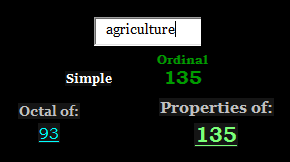"Planting" = 93 (Ordinal)
"Harvest" = 93 (Ordinal)

Every angle of Octagon (double square) is 135°
Magic square of Saturn sums to 45 - 45th triangular number is 1035 - note that Square and Circle are both 360°
Three hundred sixty = 227

Octagon and squares are frequently used symbols,  octagon can be found on top of a police hat also UFC fights happen in Octagon. These shapes are generally used to represent Base consciousness in Freemasonry, that's why a lot of the Freemasonic symbolism includes Chess board, often they will depict someone on the chess board meaning that he doesn't have higher knowledge or  doesn't know right from wrong, it represents world of duality, one who is standing on the square has no higher knowledge, and that's why a lot of the pictures have Ladder(44) going up towards the stars - Spiritual = 44In Aleister Crowley's Tarot deck of Thoth (based on Golden Dawn Tarot), the last card belongs to SaturnThe Universe-Key 21-, is the last of the Major Arcana and is the Feminine Compliment to the 0-The Fool (Aquarius - Ruled by Saturn).. The Universe is attributed to the Hebrew letter Tau and since this card is compliment to the Fool (letter Aleph),  the two together spell the word Ath, which means Essence. The Fool is the beginning and the Universe is the end. The consensus is that since everything came from nothing, the end of everything is the return to nothing.

"Saturn" = 1+1+2+3+9+5 = 21 (Reduced)
"XXI" = 6+6+9 = 21 (Reduced)

The card is attributed to the Hebrew letter Tau (Tav)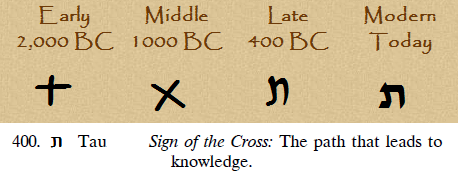Now check this outX = 144 in Sumerian system, 144 decimal places of Pi added together sum to 666
Saturn's supposed gravity - 10.44 m/s² - 144 is 12th Fibonacci number , also we have 1440 minutes in a day
It's very interesting that 144th triangular number is 10440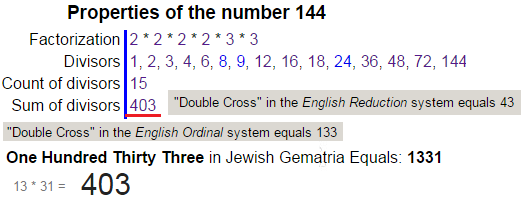Saturn is known as Time keeper - "Time" = 100+9+30+5 = 144 (Jewish), "Clock" = 3+12+15+3+11 = 44 (Ordinal)

Saturn is sometimes depicted with, Hourlgass
"Hourglass" = 8+6+3+9+7+3+1+1+1 = 39 (Reduced)
"Timekeeper" = 100+9+30+5+10+5+5+60+5+80 = 309 (Jewish)
"God of time" = 61 + 56 + 144 = 261 (Jewish)Pronounce letter H, and pronounce 'Eight' and you see why H = 8
Hourglass starts with H for a good reason, adding 2 lines to X we get ugly looking 8 or Hourglass
Also letter H is perfectly balanced, like number 8Sun is crucified at winter Solstice which takes place on December 21st/22nd

Saturn is the only month that rules 2 signs in a row

Sign of Capricorn spans from 12/21 to 1/19, which is already very interesting (119 = God Saturn, Star of David = 119)
Aquarius spans from 1/19 to 2/18
In the ancient times, March (Martius) was the first month of the year, which makes more sense, but what's interesting with the modern calendar is that we start the new year with the first 2 months of the year ruled by Saturn, and our year starts just after the sacrifice of his Son (Sun)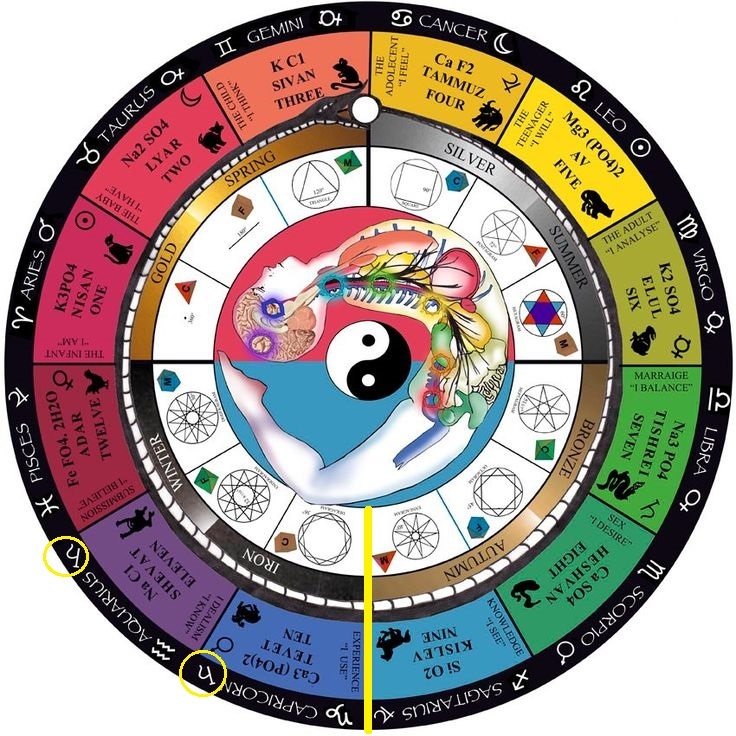Jupiter rules 2 signs that are on the left and right of Aquarius and Capricorn (Jupiter is the closest planet to Saturn)

Pisces and Sagittarius are ruled by Jupiter, which also connects to Jesus (Jupiter is the planet that rules Pisces, and when the Jesus story was written we were in the Age of Pisces which the reason for the fish symbolism surrounding it
(Roman) Jupiter is Zeus in Greek mythology (Je-Zeus = Jesus)
Jesus made Saint Peter the first pope in AD 33 supposedly, Saint Peter = Ju-PeterThis symbol is called "Ichthys"
"Ichthys" = 9+3+8+2+8+7+10 = 47 (S Exception)
Jesus = 74, Christian = 47